# 3j-symbol

In physics and mathematics, Wigner 3-jm symbols, also called 3j symbols, are related to the Clebsch-Gordan coefficients of the groups SU(2) and SO(3) through$\begin{pmatrix} j_1 & j_2 & j_3\\ m_1 & m_2 & m_3 \end{pmatrix} \equiv \frac{(-1)^{j_1-j_2-m_3}}{\sqrt{2j_3+1}} \langle j_1 m_1 j_2 m_2 | j_3 \, {-m_3} \rangle.$

The 3j symbols show more symmetry in permutation of the labels than the corresponding Clebsch-Gordan coefficients. Exactly as is true for the Clebsch-Gordan coefficients, the j-values are positive and either integral: (0, 1, 2,..) or half-integral: (1/2, 3/2, 5/2, ...).

## Note on phases

All 3j symbols are real, which means that the value of n in overall phase factors of the kind: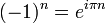$(-1)^n = e^{i\pi n}\;$

must be integral, otherwise exp[iπn] would not be on the real axis in the complex plane. For half-integral n the phase factor is purely imaginary and a 3j symbol containing the factor would be too, so that half-integral values do not appear as powers of −1. The overall powers of −1 are odd or even integral numbers. The following relation (n integral) seems not to be known to everyone contributing to the corresponding article on Wikipedia:$(-1)^n = (-1)^{-n}= \frac{1}{(-1)^n}= \begin{cases} \;\;1 &\hbox{if}\quad n\quad \hbox{even}\\ -1 &\hbox{if}\quad n\quad \hbox{odd}\\ \end{cases}$

Note that the expression$j \pm m\quad\hbox{with}\quad -j \le m \le j$

is necessarily integral, since m runs in unit steps and jmmax = j+mmin =0. Likewise j1j2±m3 is integral [±m3 = ±(−m1m2)].

##  Inverse relation

The inverse relation—the Clebsch-Gordan coefficient given by a 3j symbol—can be found by noting that j1 - j2 - m3 is an integral number and making the substitution$m_3 \rightarrow -m_3$$\langle j_1 m_1 j_2 m_2 | j_3 m_3 \rangle = (-1)^{j_1-j_2+m_3}\sqrt{2j_3+1} \begin{pmatrix} j_1 & j_2 & j_3\\ m_1 & m_2 & -m_3 \end{pmatrix}.$

##  Symmetry properties

The symmetry properties of 3j symbols are more convenient than those of Clebsch-Gordan coefficients. A 3j symbol is invariant under an even permutation of its columns:$\begin{pmatrix} j_1 & j_2 & j_3\\ m_1 & m_2 & m_3 \end{pmatrix} = \begin{pmatrix} j_2 & j_3 & j_1\\ m_2 & m_3 & m_1 \end{pmatrix} = \begin{pmatrix} j_3 & j_1 & j_2\\ m_3 & m_1 & m_2 \end{pmatrix}.$

An odd permutation of the columns gives a phase factor: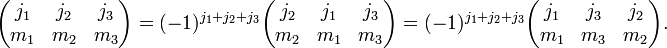$\begin{pmatrix} j_1 & j_2 & j_3\\ m_1 & m_2 & m_3 \end{pmatrix} = (-1)^{j_1+j_2+j_3} \begin{pmatrix} j_2 & j_1 & j_3\\ m_2 & m_1 & m_3 \end{pmatrix} = (-1)^{j_1+j_2+j_3} \begin{pmatrix} j_1 & j_3 & j_2\\ m_1 & m_3 & m_2 \end{pmatrix}.$

Changing the sign of the m quantum numbers also gives a phase: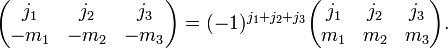$\begin{pmatrix} j_1 & j_2 & j_3\\ -m_1 & -m_2 & -m_3 \end{pmatrix} = (-1)^{j_1+j_2+j_3} \begin{pmatrix} j_1 & j_2 & j_3\\ m_1 & m_2 & m_3 \end{pmatrix}.$

##  Selection rules

The Wigner 3j is zero unless m1 + m2 + m3 = 0, j1 + j2 + j3 is integer,$|m_i| \le j_i$ and$|j_1-j_2|\le j_3 \le j_1+j_2$.

##  Scalar invariant

The contraction of the product of three rotational states with a 3j symbol,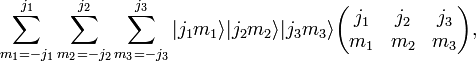$\sum_{m_1=-j_1}^{j_1} \sum_{m_2=-j_2}^{j_2} \sum_{m_3=-j_3}^{j_3} |j_1 m_1\rangle |j_2 m_2\rangle |j_3 m_3\rangle \begin{pmatrix} j_1 & j_2 & j_3\\ m_1 & m_2 & m_3 \end{pmatrix},$

is invariant under rotations.

##  Orthogonality Relations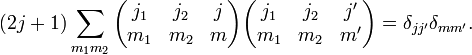$(2j+1)\sum_{m_1 m_2} \begin{pmatrix} j_1 & j_2 & j\\ m_1 & m_2 & m \end{pmatrix} \begin{pmatrix} j_1 & j_2 & j'\\ m_1 & m_2 & m' \end{pmatrix} =\delta_{j j'}\delta_{m m'}.$$\sum_{j m} (2j+1) \begin{pmatrix} j_1 & j_2 & j\\ m_1 & m_2 & m \end{pmatrix} \begin{pmatrix} j_1 & j_2 & j\\ m_1' & m_2' & m \end{pmatrix} =\delta_{m_1 m_1'}\delta_{m_2 m_2'}.$